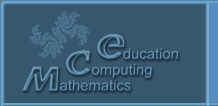Русский
 ! Вопросы и ответы

# Abstracts

## Teaching mathematics by using webMathematica

Vorob'ev E.M., Nikishkin V.A.

Higher Mathematics Chair, MESI, Nezhinskaya 7, Moscow, RF, 119501

2 pp. (accepted)

WebMathematica is a web interface for the well-known software system for doing symbolic, graphical and numeric calculation called Mathematica. The usage of Mathematica for teaching requires its licensed installation on the computers of display classes. So Mathematica is mainly intended for full-time students while webMathematica being on-line system can serve as a basis for distant education. Teaching by using webMathematica relies on interactive electronic tutorials developed by the authors.

We use webMathematica site http://webmath.mesi.ru for teaching mathematical disciplines for the past six years. There are sixteen interactive tutorials in Calculus, Linear Algebra and Differential Equations on this site. The methods of teaching math by using the tutorials is the content of our presentation.

The main advantage of the tutorials application is visualization and automation of the routine calculations. Visualization is a (dynamic) graphical or geometric representation of the mathematical objects aimed at developing an intuitive, holistic understanding of mathematical concepts and facts facilitates their verbal description. The computing automation enables teachers and students to discuss important or applied problems that is impossible within the traditional technology of teaching.

© 2004 Designed by Lyceum of Informational Technologies №1533